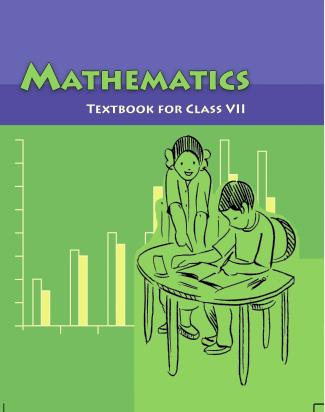1. /
2. CBSE
3. /
4. Class 07
5. /
6. Mathematics
7. /
8. NCERT Solutions for Class...

# NCERT Solutions for Class 7 Maths Exercise 3.4### myCBSEguide App

Download the app to get CBSE Sample Papers 2023-24, NCERT Solutions (Revised), Most Important Questions, Previous Year Question Bank, Mock Tests, and Detailed Notes.

NCERT solutions for Maths Data Handling## NCERT Solutions for Class 7 Maths Data Handling

###### Question 1.Tell whether the following is certain to happen, impossible can happen but not certain.

(i) You are older today than yesterday.

(ii) A tossed coin will land heads up.

(iii) A die when tossed shall land up with 8 on top.

(iv) The next traffic light seen will be green.

(v) Tomorrow will be a cloudy day.

(i) It is certain to happen.

(ii) It can happen but not certain.

(iii) It is impossible.

(iv) It can happen but not certain.

(v) It can happen but not certain.

NCERT Solutions for Class 7 Maths Exercise 3.4

###### Question 2.There are 6 marbles in a box with numbers from 1 to 6 marked on each of them.

(i) What is the probability of drawing a marble with number 2?

(ii) What is the probability of drawing a marble with number 5?

Total marbles from 1 to 6 marked in a box = 6

(i)The probability of drawing a marble with number 2.

{tex} \Rightarrow {/tex} P (drawing one marble) = {tex}\frac{1}{6}{/tex}

(ii)The probability of drawing a marble with number 5.

{tex} \Rightarrow {/tex} P (drawing one marble) = {tex}\frac{1}{6}{/tex}

NCERT Solutions for Class 7 Maths Exercise 3.4

###### Question 3.A coin is flipped to decide which team starts the game. What is the probability that your team will start?

A coin has two possible outcomes Head and Tail.

Probability of getting Head or Tail is equal.

{tex}\therefore {/tex} P (Starting game) = {tex}\frac{1}{2}{/tex}

## NCERT Solutions for Class 7 Maths Exercise 3.4

NCERT Solutions Class 7 Maths PDF (Download) Free from myCBSEguide app and myCBSEguide website. Ncert solution class 7 Maths includes text book solutions from Class 7 Maths Book . NCERT Solutions for CBSE Class 7 Maths have total 15 chapters. 7 Maths NCERT Solutions in PDF for free Download on our website. Ncert Maths class 7 solutions PDF and Maths ncert class 7 PDF solutions with latest modifications and as per the latest CBSE syllabus are only available in myCBSEguide.

## CBSE app for Students

To download NCERT Solutions for Class 7 Maths, Social Science Computer Science, Home Science, Hindi English, Maths Science do check myCBSEguide app or website. myCBSEguide provides sample papers with solution, test papers for chapter-wise practice, NCERT solutions, NCERT Exemplar solutions, quick revision notes for ready reference, CBSE guess papers and CBSE important question papers. Sample Paper all are made available through the best app for CBSE students and myCBSEguide website.### Test Generator

Create question paper PDF and online tests with your own name & logo in minutes.### myCBSEguide

Question Bank, Mock Tests, Exam Papers, NCERT Solutions, Sample Papers, Notes